# WEBWORK EFFECTIVENESS IN RUTGERS CALCULUS

### by Chuck Weibel and Lew Hirsch (July, 2002)

• WeBWorK Conclusions
• What WeBWorK is; Grouping by Class Format
• WeBWorK is the most significant predictor
• The Final Exam versus Precalculus Placement
• WeBWorK and placement scores as predictors
• Factor Analysis
• Appendix: Statistical Justification of Regressions
• Other effectiveness studies

In Spring 2001, the Rutgers mathematics department introduced a computer-based homework system into its non-science calculus course, Math 135. This system used WeBWorK, which was developed with NSF financing at the University of Rochester. Its introduction at Rutgers was made possible by a Rutgers Internal Technology Initiative, funded by a grant from the Mellon Foundation.

In this study, we try to measure how effective WeBWorK was in improving learning, measured by the students' performance in calculus. Our procedure was to treat the Fall 2001 course as a controlled experiment, using the score on the common final exam as a measure of performance. The final exam scores ranged from 0 to 200 points.

This was possible because the transition to WeBWorK was gradual, and the course is coordinated to ensure a common syllabus as well as a common final exam. During the Fall 2001 semester, about two-thirds of the 1334 students were in sections requiring weekly WeBWorK assignments. The selection of which sections should require WeBWorK was invisible to the students, and was somewhat random. This made it possible to treat the non-WeBWorK sections as a control group. The first hypothesis being tested was that WeBWorK is effective in raising scores on the final, i.e., in enhancing student performance.

It quickly became apparent that three sub-populations of students had different profiles. First-year students made up the largest sub-population (62% of everyone); they responded much better to WeBWorK than upper-class students. A second type of student, which we shall refer to as "non-repeaters," consisted of upper-class students who were taking calculus for the first time. The third sub-population consisted of students who were repeating the course; we shall refer to them as the "repeaters."

Table 1 displays the average final exam scores for the control group and the WeBWorK sections, along with a breakdown by sub-populations. The corresponding letter grades are given as a guidline. Over all students (line 1 in the table), the average final was 8 points higher in WeBWorK sections than in the control sections, which is significant at the 95% confidence level, because the standard error of the mean is about 2.1. However, the control/WeBWorK differences within the sub-populations were not statistically significant.

Type of studentControl SectionsWeBWorK Sections Std. Error WW>80% WW<50%
All students 130.7 (C+)138.1 (C+) 2.1156.5 (B)107.2 (C)
First-year students147.4 (B)150.1 (B) 2.5161.1 (B+)119.8 (C)
Non-repeaters 114.3 (C)114.4 (C) 4.0138.7 (B-) 95.1 (D)
Repeaters 102.1 (C-)107.4 (C-) 6.1110.4 (C) 97.5 (D)

Table 1: Mean FINAL Exam Scores, for Control and WeBWorK groups

On the other hand, if we restrict to students who did at least 80% of all WeBWorK assignments, the averages rise dramatically. Similarly, if we restrict to students who did at most 50% of all WeBWorK assignments, the averages drops dramatically (except for repeaters). One way of stating these observations is this:

Just being in a WeBWorK class is not enough; students must actually do the assignments in order to reap the benefit on the final. However, there was a statistically significant difference of half a letter grade between students in the control group, and students who were not only assigned WeBWorK problems but attempted them.

### Findings

The availability of WeBWorK does not help students unless they use it. Students in WeBWorK sections did slightly better than the control group. However, within WeBWorK sections, students who did over 80% of the WeBWorK problems performed dramatically better (by a full letter grade) than those who did less than half of the WeBWorK problems. There was no significant distinction between the percentage of WeBWorK problem sets attempted and the percentage of problems solved correctly.

In WeBWorK sections, the dominant predictors of final exam score in WeBWorK sections were the Precalculus placement score and WeBWorK score. In the Control group, the dominant predictor of final exam score was the Precalculus placement score. The effect of WeBWorK varied dramatically from population to population.

There was a weak correlation between WeBWorK performance and placement scores (.23 for first-year students, .14 for non-repeaters and .05 for repeaters). This suggests that a high precalculus skill level helps students do well on WeBWorK, but it cannot be used to predict how well.

### Grouping by Class Format

In Fall 2001, we had 1334 students take the common final exam, and had more complete data for 1159 students. Students were taught in two class formats, with and without WeBWorK. The regular class format consisted of two 80-minute lectures and one 55-minute recitation each week. The "practicum" class format consisted of small classes, two 80-minute lectures and two 55-minute recitations each week, one for homework and one for workshops.

The number of students taking the final exam is given by table 2. We divided the students into four groups, according to the class format. The control group ('C') consisted of 296 students (25%) in the regular class format, with no web-based homework. The WeBWorK group ('W') consisted of 715 students (62%) in the regular class format, with web-based homework. There was a third group ('M') of 72 students (6%) who switched to WeBWorK in the middle of the semester, and a fourth group ('S') of 76 students (7%) in the small practicum sections, who did no web-based homework.

Type of Student ALL CWMS
First-year students 857 2025534458
Non-repeaters286 911552515
Repeaters102 40 51 8 3
Unknown status89 35 4851
totals1334 3688078277

Table 2: Number of students, by type and group
C=control group; W=WeBWorK sections; M=switched mid-semester; S=small practicum

For the purposes of this report, the performance of mixed group M and the small practicum group S were not relevant. In addition, their small size meant that little could be concluded about their behaviour. Therefore the bulk of this report will focus upon the Control Group C and the WeBWorK group W.

Here is a table showing how differently these sub-populations performed, both on WeBWorK and on the FINAL exam. TOTAL is the percentage of all WeBWorK problems completed, and the FINAL score is out of 200 points. We have attached letter grades to the FINAL scores for calibration purposes; below 90 points was failing.

Type of studentnumberTOTAL %FINAL
All students936 (100%)63.9 138.1 (C+)
First-year students583 (62%)75.8 147.4 (B)
Upper-class non-repeaters211 (23%)46.6 114.5 (C)
Unknown status68 (7%)47.2 110.2 (C)
Repeaters74 (8%)34.4 102.1 (C-)

Table 3: WeBWorK TOTAL and FINAL Exam means
(students in WeBWorK sections)

At one extreme we have first-year students; they did most of the WeBWorK problems and also did well on the final exam. At the other extreme we have repeaters; they did only a third of the WeBWorK on average, and also did poorly on the final exam.

### What WeBWorK is

WeBWorK is a web-based homework checker, allowing calculus students to get feedback on weekly problem sets. Dozens of math departments in the United States use some form of WeBWorK, including Rutgers. The original model of WeBWorK was created in 1996 at the University of Rochester.

Each week, students log on to WeBWorK and are given a set of homework problems. The problems are similar but slightly different for each student. They can collaborate in finding solutions, but they still need to answer their own individual problems. When they are ready, they enter their answers into the computer. WeBWorK immediately tells students if their answers are correct, but does not tell them the correct answers. They are allowed to try to answer the same question again, until they get it right. Most students get a correct answer within 3 tries; it is rare for a student to try more than 10 guesses, and then get the right answer.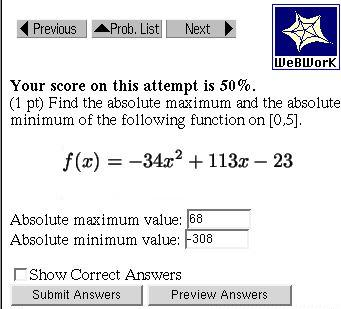Sample WeBWorK problem.
The score is 50% because one answer is wrong.

To measure WeBWorK performance, we used a variable called TOTAL, which is the percentage of all WeBWorK problems solved correctly. Although we had detailed information about each of the ten WeBWorK problem sets, all of it was highly correlated. The correlation between the number of sets attempted and TOTAL was a remarkable .944; the correlation between TOTAL and any individual set ranged from .70 to .88, which is also very high. With a few exceptions, the correlation between scores on any two sets ranged from .63 to .75. Our analysis of variance showed that TOTAL was a more significant predictor of final exam score than the number of attempts, or other combinations we tried.

It is important to understand that most students in WeBWorK sections did most of the WeBWorK problems. About 11% of students in these sections did not attempt WeBWorK at all; many of these dropped the course, and hence did not take the final exam. (See bar chart 4 below.) Excluding these, about a third had a WeBWorK TOTAL of under 50%, a third had a TOTAL between 50 and 90%, and a third had a TOTAL over 90%.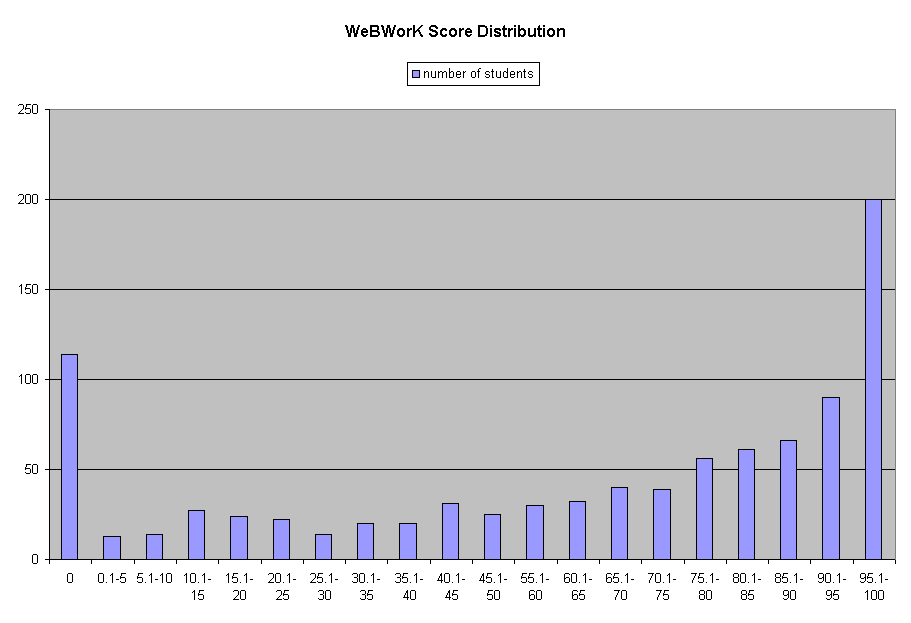Bar Chart 4: Distribution of WeBWorK TOTAL scores

Do the better prepared students do better at WeBWorK? To answer this question, we did an analysis of variance, using the WeBWorK TOTAL as dependent variable. Among the 380 first-year students in WeBWorK sections, the best numerical predictors were High School rank and Precalculus placement score. However these predicted only 9% of the variance in the WeBWorK totals. As illustrated in Tables 1 and 3, the best predictor was the student's history: first-year, upper-class non-repeater or repeater.

## WeBWorK is the most significant predictor

An analysis of variance was performed on each sub-population within WeBWorK sections, in order to find the most significant predictors of performance on the final exam. The variables we considered included: WeBWorK score, Precalculus placement, SAT scores (Math and Verbal), High School rank, and gender.

For the entire population, the most significant predictor was the WeBWorK TOTAL, with a regression of FINAL = 82.7 + (.81)TOTAL. According to the R-squared value, this accounted for 38% of the variability in the data. Although this predicts a swing of 3-1/2 letter grades, from 83 points (F) for no WeBWorK to 164 points (B+) for all WeBWorK, our more detailed analysis below shows that this is not a good interpretation.

In fact, the data suggests a quadratic relationship between the WeBWorK TOTAL and the FINAL Exam score, with the best-fitting curve being concave up. That is, students who do less than 50% of the WeBWorK get less "marginal" benefit on the final (improvement from doing one more problem) than students who do over 80% of the WeBWorK. To estimate this relationship, it is convenient to express the WeBWorK score as a fraction T (from 0 to 1) rather than as TOTAL, which is a percentage. That is, T = TOTAL/100. (See figure 5.) The best-fitting quadratic polynomial to the data for all 802 students was:

FINAL = 90 + 30*T + 43*T^2.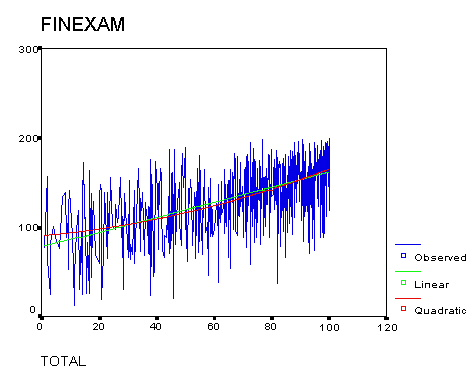Figure 5: Final Exams versus WeBWorK TOTAL (best linear and quadratic fits, all students)

This means that the difference between doing one WeBWorK set (T=.1) and two sets (T=.2) would be only 4 points on the final (from 93 to 97), while the difference between doing 9 sets and all 10 WeBWorK sets would be 12 points on the final (from 151 to 163).

#### WeBWorK for First-year Students

For the first-year students, the most significant predictor was the WeBWorK TOTAL, with a regression of FINAL = 103.8 + (.62)TOTAL. According to the R-squared value, this accounted for 25% of the variability in the data. Although this predicts a swing of 3 letter grades from 104 points (C) for no WeBWorK to 176 points (A) for all WeBWorK, this is not a good interpretation either. (See figure 5a.)Figure 5a: Final Exams versus WeBWorK TOTAL (first-year students)

Note that the data is skewed to the right, with an average WeBWorK TOTAL of 75.8 and a standard deviation of 28.5.

#### WeBWorK for Upper-class Non-repeaters

Another interesting population consisted of upper-class students who were taking calculus for the first time at Rutgers, students we refer to as non-repeaters. This was a more diverse group. Only 11% of these students had originally placed into calculus; presumably they didn't take calculus in their first year because of other course priorities. Another 43% placed into Precalculus, and 32% placed below precalculus; these students had therefore already taken some college-level mathematics courses, at Rutgers or elsewhere. The remaining 14% consisted of transfer students who did not take the placement tests.

For upper-class students taking calculus for the first time, the WeBWorK TOTAL was also the most significant predictor of the final exam score, accounting for 34% of the data variability. The least-squares fit for this group was FINAL = 68.3 + (.77)TOTAL. Although this predicts a swing of 3 letter grades from 68 points (F) for no WeBWorK to 145 points (B) for all WeBWorK, this is not a good interpretation either.
Figure 5b is a scatterplot showing the least-squares fit.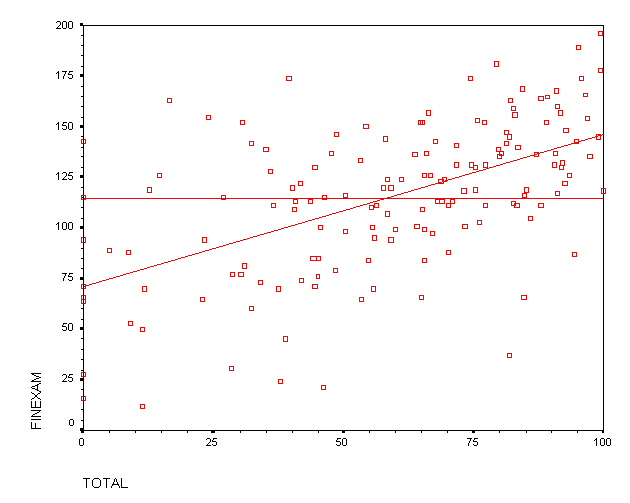Figure 5b: Final Exams versus WeBWorK TOTAL (upper-class non-repeating students)

#### WeBWorK for Repeaters

For students repeating calculus, there was almost no connection between WeBWorK score and performance on the final. In fact, the least-squares fit is almost meaningless, accounting for only 4% of the vaiance in the data. This is vividly illustrated by the scatterplot in figure 5c: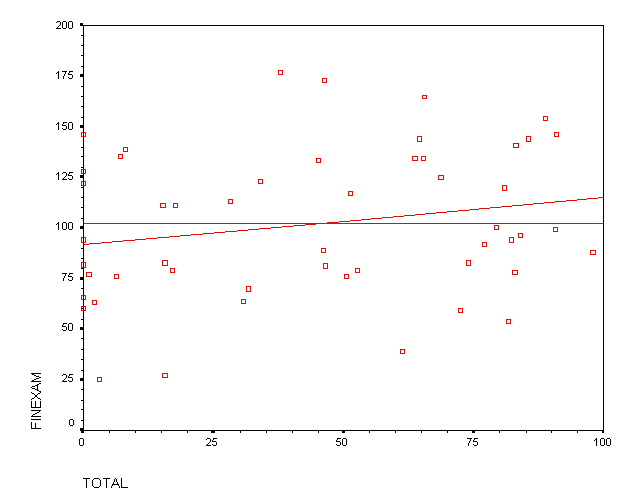Figure 5c: Final Exams versus WeBWorK scores (students repeating calculus)

## The Final Exam versus Precalculus Placement

We next performed a comparison to our previous study [WH] (in 2000) of teaching effectiveness in this class. The performance of each student was measured by their score, FINAL, on the common final exam. This was a 3-hour exam given at the end of the semester, and graded on a scale of 0-200 points. The median FINAL score was 128. We found that the overall distribution of FINAL scores was very similar to the distribution described in our previous study [WH].

Our previous study had shown the importance of the Precalculus placement score, PCAL, which is administered to all incoming Rutgers students. PCAL is measured on a scale of 0-35 points; incoming students cannot take calculus unless PCAL was at least 21 points. As in our previous study, we included many other variables (gender, SAT scores, etc.) in an analysis of variance, and recovered our previous finding that the other variables were not significant.

Here are the results of our regressions between PCAL and FINAL. These results are consistent with our previous findings. See the Appendix for a justification of these regressions.

Type of Student/Group PCALMSATFINAL FINAL vs PCAL
All students22.4622135.4 F = 64.1 + (3.19)PCAL
Control Group C21.6617130.7 F = 64.0 + (3.11)PCAL
WeBWorK Group W22.8624138.1 F = 64.0 + (3.25)PCAL
Small Practicum Group S23.7635136.8 F = 44.3 + (3.89)PCAL
First-year students 26.3649149.1 F = 46.2 + (3.93)PCAL
First-year (control group C) 26.0649147.4 F = 46 + (3.9)PCAL
First-year (WeBWorK group W) 26.4648150.1 F = 49 + (3.7)PCAL
Non-repeaters13.9571113.4 F = 80.8 + (2.20)PCAL
Non-repeaters (control group C)13.9574114.3 F = 81.9 + (2.16)PCAL
Non-repeaters (WeBWorK group W)13.9569114.5 F = 80.3 + (2.31)PCAL
Repeaters18.3584104.2 F = 61.6 + (2.27)PCAL
Repeaters (control group C)19.0573107.4 F = 39.8 + (3.38)PCAL
Repeaters (WeBWorK group W)17.6585102.1 F = 63.2 + (2.27)PCAL

Table 6: Precalculus Placement versus Final Exam

This data shows no statistically significant difference between students in WeBWorK sections and students not in WeBWorK sections, even those with similar precalculus skills. One explanation for this is that just being in a WeBWorK section did not mean that a student actually used the WeBWorK.

To test the effect of WeBWorK we had to combine the placement score (PCAL) with the WeBWorK score (TOTAL).

## WeBWorK and placement scores as predictors

To determine the most significant predictors of final exam score, we did an analysis of variance using the 522 students in WeBWorK sections for which we had complete data. This analysis indicated that the two most significant predictors of final exam score were: (a) the WeBWorK TOTAL, and (b) the precalculus placement score PCAL. For the 709 students for which we had this data, the best fit was:

FINAL = 41.5 + (2.32)PCAL + (.61)TOTAL

This model accounted for 54% of the variance in the data (as measured by the R-squared statistic); adding more variables did not appreciably affect the variance.

#### Predicting first-year student performance

For the first-year students who were in WeBWorK sections, TOTAL and PCAL were also the most significant predictors. Among the 524 first-year students for which we had this data, these variables accounted for 43% of the variance in the data (as measured by the R-squared statistic). The least squares fit was:

FINAL = 18 + (3.26)PCAL + (.59)TOTAL

For an average student with a placement score of 26.0, this predicts a FINAL score of between 103 (C-) and 162 (B+), depending on the WeBWorK TOTAL.

In order to compare this model with the data from the control group, we adjusted the final score by subtracting off the best fit for all first-year students from Table 6, setting:

ADJ.FIN = FINAL - (46.2 + 3.93*PCAL).

To illustrate the lack of gender difference in this model, the scatterplot of the adjusted final versus WeBWorK TOTAL is given in figure 7 by gender: the green data represents the men and the red data represents the women in our sample of first-year students.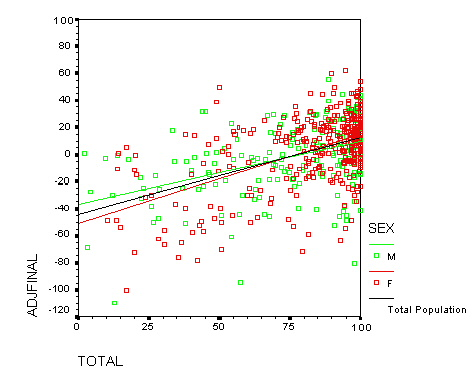Figure 7: Adjusted Final Exams versus WeBWorK TOTAL (First-year students, by gender)

The data cluster in the upper right is a very noticeable feature. It reflects the fact that most first-year students did most of the WeBWorK problems. It also reflects the fact that the effect of WeBWorK is quadratic. To illustrate this quadratic nature, and also to spread out the data somewhat, figure 7a plots the adjusted final versus the T^2, the square of the WeBWorK total (expressed as a fraction). As in figure 6, we have grouped the data by gender.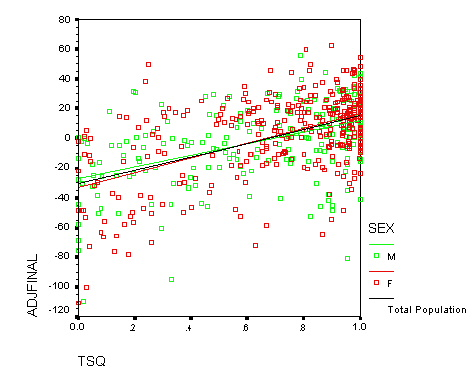Figure 7a Adjusted Final Exams versus squared WeBWorK scores (first-year students, by gender)

#### Predicting upper-class Non-Repeater's performance

For the upper-class non-repeating students who were in WeBWorK sections, PCAL and TOTAL were also the most significant predictors. Among the 108 non-repeating students for which we had this data, these variables accounted for 39% of the variance in the data (as measured by the R-squared statistic). The least squares fit was:

FINAL = 51 + (1.45)PCAL + (.71)TOTAL

For an average non-repeating student with a placement score of 13.9, this predicts a FINAL score of between 71 (F) and 142 (B), depending on the WeBWorK TOTAL.

A similar least-squares fit, with the Rutgers Precalculus Placement score replaced by the Math SAT score, gave almost identical results. Perhaps this is not so surprising, since the correlation between Math SAT and placement scores was a high .66.

In order to visualize the effect of WeBWorK upon final exam scores, we adjusted the final by subtracting off the best fit for all upper-class non-repeating students from Table 6, setting:

AD.FIN2 = FINAL - (80.8 + 2.2*PCAL).

For an average student with a placement score of 14.0, this predicts a FINAL score of between 111 (C) and 162 (B+), depending on the WeBWorK TOTAL.Figure 8b: Adjusted Final Exams versus WeBWorK TOTAL (upper-class non-repeating students)

Visually, there is a clear relation between the WeBWorK score and the adjusted final exam score, especially for students who do at least half of the WeBWorK assignments. In fact, this scatterplot is not much different than Figure 5b.

#### Predicting performance of Repeating students

There were only 51 students in WeBWorK sections that were repeating the course. Of these, we had complete data (High School rank, etc.) for only 30 of these students. An analysis of variance showed that the most significant predictor of final exam scores was the verbal SAT score (accounting for only 22% of the variance), followed by the WeBWorK TOTAL and the Precalculus Placement score, PCAL. We believe that the appearance of the new variable (verbal SAT) is due primarily to the small sample size, since it is difficult to see the mechanism by which the verbal skill level would play a significant role on the final exam.

In order to plot final exam scores versus WeBWorK scores, we adjusted the final exam score by substracting off the best placement fit. That is AD.FIN2 = FINAL - (61.6 + 2.2*PCAL). A visual inspection of Figure 8c (which resembles Figure 4c) shows that there is almost no connection between WeBWorK score and performance on the final, even after adjusting for placement scores.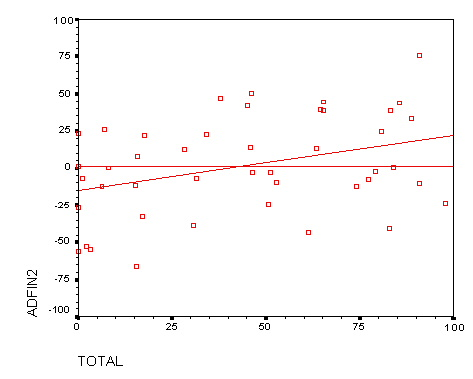Figure 8c: Adjusted Final Exams versus WeBWorK scores (students repeating calculus)

## Factor Analysis

Since there was some correlation between the various predictors of final exam score, we also did a factor analysis to determine what the most important predictive factors were. As in our earlier study, we restricted to first-year students, with the additional restriction that they did at least one WeBWorK problem, and took the final exam. We found two significant factors.

The most important factor was a combination of many variables, including the Math SAT (scaled from 200 to 800). Associated to this factor is a new predictor, which we christened "NEWP." By definition:

NEWP = 0.0582*MSAT + 0.738*PCAL + 0.635*TOTAL.

Figure 9 shows that NEWP is a slight improvement upon the predictors used in Figures 4a and 6. As before, the best fitting curve is concave up.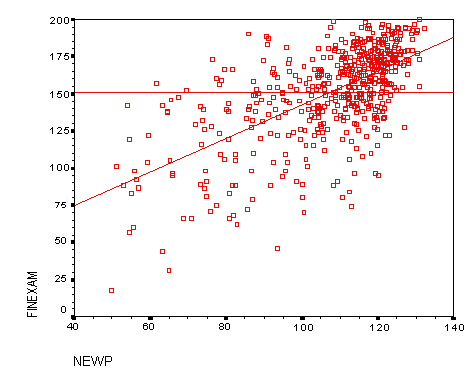Figure 9: Final Exams versus New Predictor (first-year students)

The second factor was essentially a combination of High School Rank (HSR) and the WeBWorK TOTAL:

(FINAL, MSAT, PCAL, HSR, TOTAL) = (.33,-.27,.00,.40,.50)

We may think of this factor as "hard work" because it measures performance (in High School rank, WeBWorK and on the final) without much regard to previous skill level.

## APPENDIX: Statistical Justification of Regressions

In the study, we did several linear regressions of the final exam scores FINAL using placement scores PCAL (See
Table 6). In this appendix we explain why these regressions are reasonable.

The statistical assumptions used to justify least squares regressions in one variable are that:
1. The residuals are normal.
2. Homoscedasticity holds. This means that the variance is constant across outcome levels.

Since the analyses of residuals were similar in each case, we will only describe the analysis for one case, the population of 719 first-year students in either the control or WeBWorK groups. The variable ADJFINAL = FINAL - (46.2 + 3.93*PCAL) used in the study is essentially the residuals in this case (where the least-squares fit is 46.0+3.937*PCAL). Chart A1 shows the frequency distribution for the residuals; it is close to a normal distribution with standard deviation 30, but is skewed slightly right (Skewness -1.0) and has a longer left tail (Kurtosis 1.95) than expected.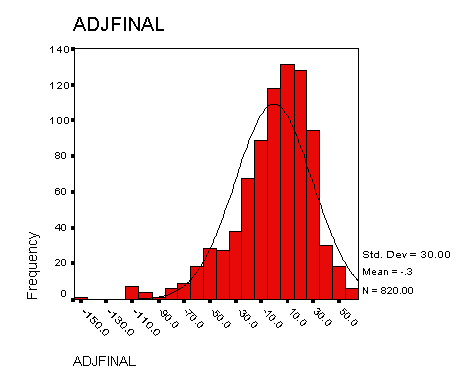Chart A1: Frequency distribution for ADJFINAL vs. a normal distribution

To check homoscedasticity, we consider the quartile-quartile (QQ) plot of the residuals against the normal distribution. It is a good fit for students within two standard deviations of zero, but breaks down for the 5% of students at the low extreme. It is somewhat truncated at the high extreme because of the cap (a perfect score of 200) on the final exam score, FINAL.Figure A2: QQ Plot comparing ADJFINAL to a normal distribution

The verification of normality and homoscedasticity for the regression of FINAL against WeBWorK score (TOTAL) is similar. Figure A3 shows the quartile-quartile (QQ) plot of the residuals against the normal distribution.Figure A3: QQ Plot comparing residuals to a normal distribution
ADJ2 is the residual for the regression of FINAL against TOTAL

For least squares regressions with two or more variables, such as the regression above of FINAL against PCAL and TOTAL, there is one additional assumption:
3. The predictor variables are linearly independent.
As we mentioned in the study, the correlation between PCAL and TOTAL was low: .23 for first-year students, .14 for non-repeaters and .05 for repeaters. So this assumption is not badly violated either.

We would like to thank Prof. S. Geller of Texas A&M University for several discussions about the statistical methods we have used in this study.

### References

[W] C. Weibel, Effectiveness of Rutgers' Calculus Formats - Part I, 1999.

[WH] L. Hirsch and C. Weibel, Effectiveness of Rutgers' Calculus Formats - Part II, 2000.

weibel @ math.rutgers.edu